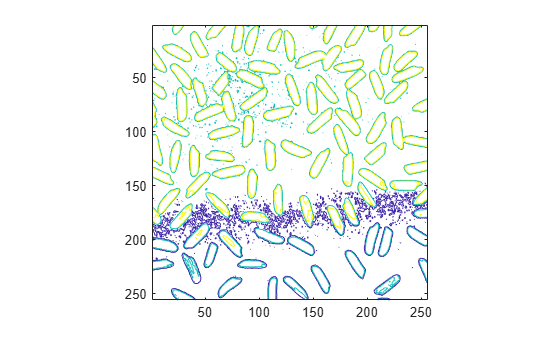Documentation

Contour Plot of Image Data

A contour is a path in an image along which the image intensity values are equal to a constant. You can create a contour plot of the data in a grayscale image using imcontour. This function is similar to the contour function in MATLAB®, but it automatically sets up the axes so their orientation and aspect ratio match the image. To label the levels of the contours, use the clabel function.

Create Contour Plot of Image Data

This example shows how to create a contour plot of an image.

Read grayscale image and display it. The example uses an example image of grains of rice.

imshow(I)Create a contour plot of the image using imcontour .

figure;
imcontour(I,3)